# opencv项目实践二（银行卡卡号识别）

## 任务说明; 实现

、思路
1、定位银行卡卡号数字区域
2、将银行卡每个卡号数字单独提取出来
3、处理模板图片，将每个模板数字单独提取出来
4、通过模板匹配来识别每个卡号

、具体代码实现
1、处理模板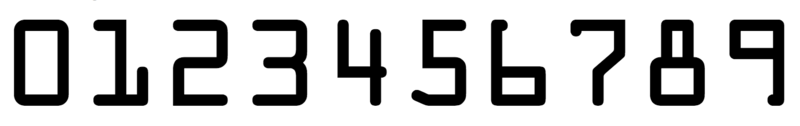``````
img_m_g = cv2.cvtColor(img_m,cv2.COLOR_BGR2GRAY)

img_m_g = cv2.threshold(img_m_g,0,255,cv2.THRESH_OTSU|cv2.THRESH_BINARY_INV)

img_m_g_con = cv2.findContours(img_m_g,cv2.RETR_EXTERNAL,cv2.CHAIN_APPROX_NONE)
img_m_g_dst = cv2.drawContours(img_m.copy(),img_m_g_con,-1,(0,0,255),2)
imshow(img_m_g_dst)
``````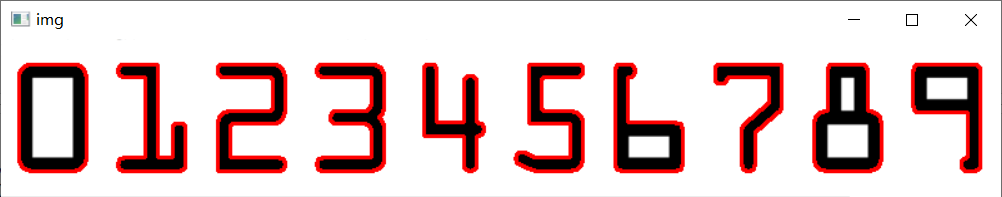``````
l1 = []
for con in img_m_g_con:
(x,y,w,h) = cv2.boundingRect(con)
l1.append(x)

(img_m_g_con,l1) = zip(*sorted(zip(img_m_g_con,l1),key=lambda x :x,reverse=False))

digits = {}
for i,con in enumerate(img_m_g_con):
(x,y,w,h) = cv2.boundingRect(con)
roi = img_m_g[y:y+h,x:x+w]

digits[i] = cv2.resize(roi,(57,88))

``````

2、处理银行卡图片

``````
img_card_gray = cv2.cvtColor(img_card,cv2.COLOR_BGR2GRAY)

kernel = np.ones((5,5))
tophat = cv2.morphologyEx(img_card_gray,cv2.MORPH_TOPHAT,kernel)
imshow(tophat)
````````````
grady =  cv2.Sobel(tophat,ddepth = cv2.CV_32F,dx=0,dy=1,ksize = 3)

````````````
kernel = np.ones((3,3))

``````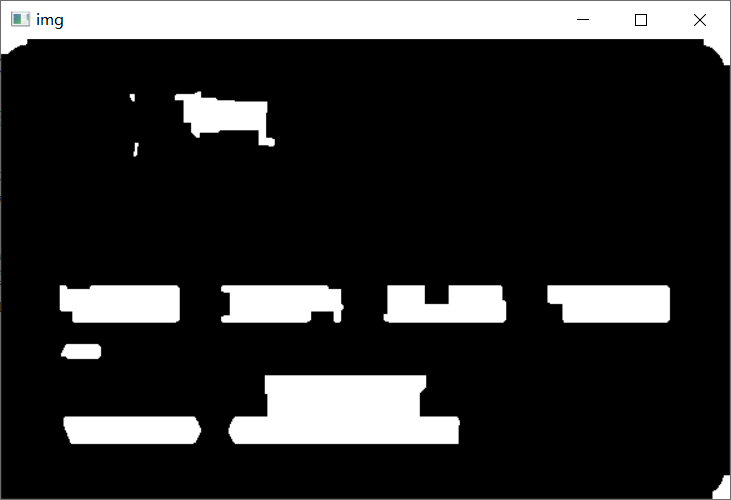``````
imshow(dst)

losc = []
(x,y,w,h) = cv2.boundingRect(con)
ar = w/h

if ar >2.4 and ar <3.5:
if w>155 or w<85:
continue
losc.append((x,y,w,h))
``````
``````
sort_losc = sorted(losc,key = lambda x :x,reverse=False)

``````
``````
img_part = []
for i in sort_losc:
x,y,w,h = i,i,i,i
con = np.array([[[x,y],[x+w,y],[x+w,y+h],[x,y+h]]])
img_part.append(img_card[y:y+h,x:x+w])
imshow(img_card[y:y+h,x:x+w])
``````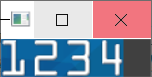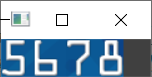``````
digital = []
def f(img):
img_gray = cv2.cvtColor(img,cv2.COLOR_BGR2GRAY)
img_2 = cv2.threshold(img_gray,0,255,cv2.THRESH_OTSU)

imgcon = cv2.findContours(img_2,cv2.RETR_EXTERNAL,cv2.CHAIN_APPROX_NONE)
new_con = []

for con in imgcon:
(x,y,w,h) = cv2.boundingRect(con)
if h>35 or w<15:
continue
new_con.append((x,y,w,h))

sort_con = sorted(new_con,key = lambda x:x)
for i in sort_con:
x,y,w,h = i,i,i,i
con = np.array([[[x,y],[x+w,y],[x+w,y+h],[x,y+h]]])
roi = img_2[y-1:y+h+1,x-1:x+w+1]

digital.append(cv2.resize(roi,(57,88)))
for i in img_part:
f(i)

``````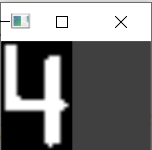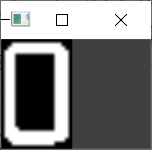...

3、模板匹配

``````
ans = []
for img_dig in digital:
now = []
for (dig,digroi) in digits.items():
res = cv2.matchTemplate(img_dig,digroi,cv2.TM_CCOEFF_NORMED)
now.append(res.item())
ans.append(now.index(max(now)))
now.clear()
print(ans)
``````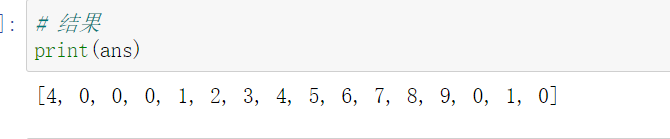cv2.matchTemplate是用于模板匹配的函数，第一个参数为待匹配图像，第二个参数为模板图像，模板图像和原图像的大小要保持一致，第三个参数为匹配方式。返回值越大，相似度越高。

1、不同格式的银行卡图片需要对参数进行幅度较大的改动。
2、没有考虑银行卡图片倾斜的情况，如果输入的银行卡图片在输入的图片中只是倾斜的，可以使用透视变换进行校正，具体做法可以参考上一篇blog（答题卡识别）

Original: https://blog.csdn.net/qq_44805233/article/details/123192913
Author: 棒子胡豆
Title: opencv项目实践二（银行卡卡号识别）

## Title: 语音合成数据解决方案助您获取专属AI声音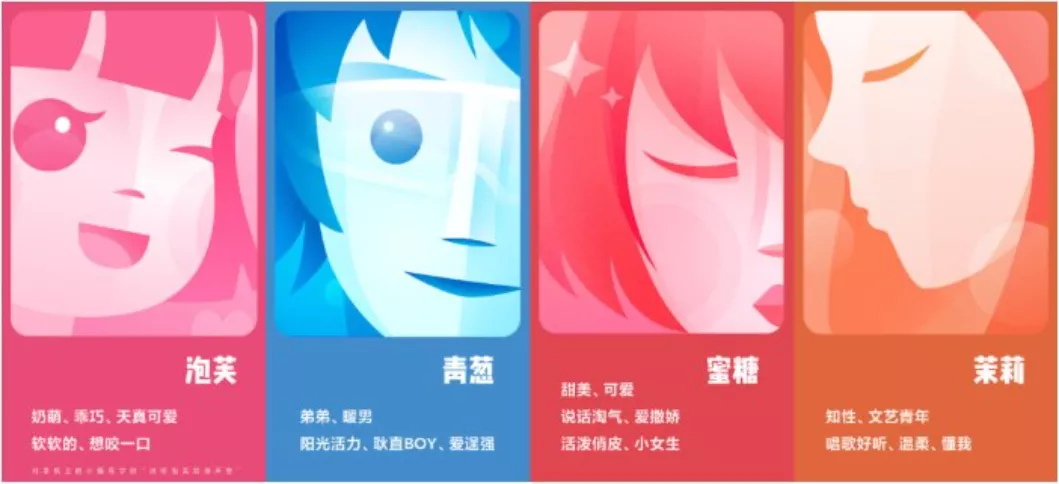01

TTS涉及声学、语言学、数字信号处理、计算机科学等多个学科技术，是信息处理领域的一项前沿技术，解决的主要问题就是如何将文字信息转化为可听的声音信息，即让机器像人一样开口说话。02

01

0203

04

03

· 智能客服· 有声读物· 车载场景· 音乐合成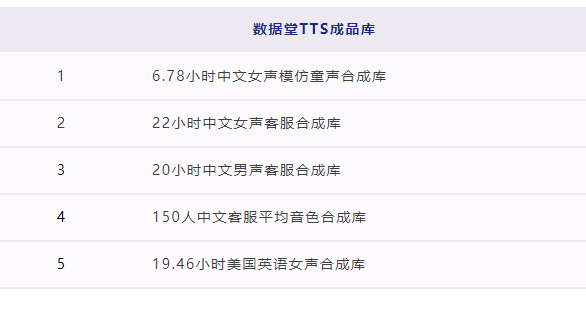Original: https://blog.csdn.net/weixin_44532659/article/details/122620574
Author: 数据堂官方账号
Title: 语音合成数据解决方案助您获取专属AI声音

# 2012/12/28（第4章 类）

## 1 用var声明局部变量

p109

``````Employee harry = new Employee("Harry Hacker");
``````

``````var harry = new Employee("Harry Hacker");
``````

## 2 null值的应用

p109

``````LocalDate birthday = null;
String s = birthday.toString();
``````

### 2.1 宽容型：

``````public Employee(String n, double s, int year, int month, int day)
{
name = Objects.requireNonNullElse(n,"unknow");//如果name值为null，则改为unknown
...

}
``````

### 2.2 严格型：

``````public Employee(String n, double s, int year, int month, int day)
{
Objects.requireNonNull(n,"name can't be null");
name = n;
...

}
``````

## 3 隐式参数和显式参数

p110

``````employee007.raiseSalary(5);
``````

## 4 封装的优点

p111

1，封装实现了getter和setter方法，防止类内的变量被随意修改，同时也可以设置getter和setter的权限等级来实现分级访问或者修改

2，同时，对于封装好的方法，如果在类内的某些参数发生了改变，我们只需要调整getter或setter函数便可保证整个代码继续可用，而不需要去找到每个可能使得代码异常的地方，如下

``````class Employee
{
private string name;

public string getName()
{
return name;
}
}
``````

``````class Employee
{
private string firstName;
private string lastName;

public string getName()
{
return firstName + " " + lastName;
}
}
``````

3，同时，getter和setter也可用于错误检查，例如setSalary可检查在设置薪资时是否为小于0的异常值

``````public void setSalary(int s)
{
if(s
``````

``````class Employee
{
private Date hireDay;
...

public Date getHireDay()
{
return hireDay;
}
...

}

public static void main(string[] arg)
{
Employee harry = ...;
Date d = harry.getHireDay();
double tenYearInMilliSeconds = 10*365.25*24*60*60*1000;
d.setTime(d.getTime() - (long) tenYearsInMilliseconds);
//let's get Harry ten years of added seniority

}
````````````class Employee
{
private Date hireDay;
...

public Date getHireDay()
{
return (Date) hireDay.clone();
}
...

}
``````

Original: https://www.cnblogs.com/luoyoucode/p/15709263.html
Author: 落悠
Title: 2021/12/28组会

## Title: 2个函数宏技巧

1.用宏调用对象函数

```#define FOR_EACH_OBSERVER(ObserverType, observer_list, func) \
do{ \
CObserverListBase<ObserverType>::Iterator it(observer_list); \
ObserverType* obs; \
while((obs=it.GetNext()) != NULL) \
obs->func; \
} while(0)
```

``````//     void NotifyBar(int x, int y) {
//       FOR_EACH_OBSERVER(Observer, observer_list_, OnBar(this, x, y));
//     }
``````

2.用宏定义函数指针来转发

```class TestDelegate
{
public:
void Test(int i)
{
i=1;
}

void Test2(int i,int j)
{
i=1;
}

void (Test22)(int i,int j)
{
//((this)->*(&TestDelegate::Test))(i);
}
};

#define __IMPLEMENT_COMSINK_FUNCTION(func, params, values) \
typedef void (T::*F##func)params;\
void Hook_##func(F##func pf##func) \
{ \
m_pf##func = pf##func; \
} \
void (func)params \
{ \
((m_pT)->*(m_pf##func))values; \
} \
private:\
F##func m_pf##func;

template<typename T>
class DelegateHandler
{
public:
DelegateHandler(T* pT,void (T::*pFunc)(int))
:m_pT(pT),m_pFunc(pFunc)
{

}

__IMPLEMENT_COMSINK_FUNCTION(OnEvent,(int a,int b),(a,b))

private:
T* m_pT;
void (T::*m_pFunc)(int);
};

```
``````TestDelegate td;
DelegateHandler dh(&td,&TestDelegate::Test);
dh.Hook_OnEvent(&TestDelegate::Test2);
dh.OnEvent(4,3);
``````

Original: https://www.cnblogs.com/Clingingboy/p/3435435.html
Author: Clingingboy
Title: 2个函数宏技巧

## 《30天吃掉那只 TensorFlow2.0》 开篇辞（Tensorflow 学习之路）

《30天吃掉那只 TensorFlow2.0 》开篇辞 🔥🔥 文章目录 《30天吃掉那只 TensorFlow2.0 》开篇辞 🔥🔥 * 一，TensorFlow2 🍎 还是 Pytorch🔥 二，K...

## [人脸算法]技术方向综述

01 人脸技术的应用 人脸硬件产品：考勤支付，安防监控，医疗美容 人脸软件产品：各类娱乐软件，如美图秀秀 02 人脸图像算法及其研究方向 人脸检测核心算法 目的：检测图像中是否存在人脸 人脸检测是目标...

## 文字识别成语音_百度Ai语音识别文字转语音

2020 04 08 语音识别-文字转语音 01前言 接上一期内容，这次利用 百度Ai 提供的语音合成api，将获取到的小说文字数据，转化为 音频数据 。需要有一个百度账号，然后再创建一个语音合成的应...

## 人工智能专题讲学：开源数据支撑下的人物与装备分析

3月19日，南京摄星智能科技有限公司CAO张侨应邀参与了某军校举办的课程专题讲学，进行了题为《开源数据支撑下的人物与装备分析》的在线专题讲座。 本次课程中，张侨结合摄星智能研发的多项产品与技术，详细介...